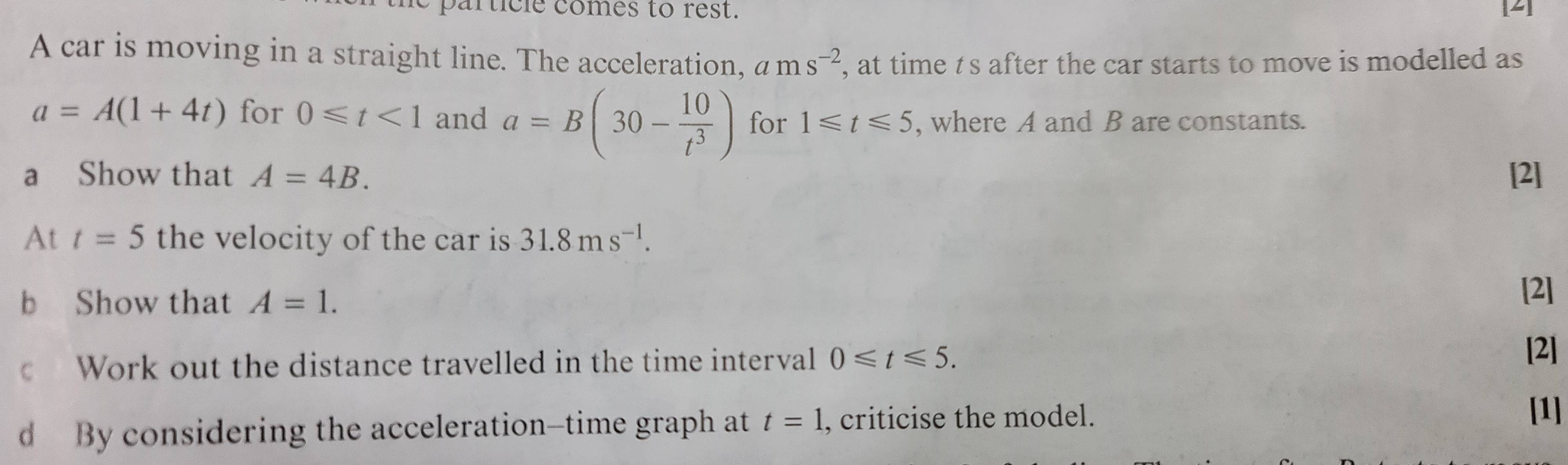# General motion in a straight line

• MHB
• Shah 72

#### Shah 72

MHBI calculated (a)
I don't know how to calculate (b)

after integrating both of them you will get this
Thank you so so so so much! That was so so helpful!

after integrating both of them you will get this
Can you also pls tell how to calculate 7(c).
I calculate s1 for interval between t=0 and t=1 and s2 for the interval between t=1 and t=5 by integrating their respective velocities. I did that and I get the ans 30m but the textbook ans is 69.2m. Pls help

For $0 \le t \lt 1$ $s= 1/2 t^2 + 2/3 t^3$ putting t=1 we get 7/6
For $1 \le t \le 5$ $v= 30/4 t + 5/4 t^{-2} - 23/4$ integrate and putting limits we get $x-7/6 = (15/4) (5^2 - 1^2) + (5/4)(1- 1/5)+ (23/4)(1-5)$ I'm getting x =63 + $7 \over 6$ So total distance = 63 + $7 \over 3$ = 65.34
Even I am also not getting the answer may be I made a calculation mistake or the answer is wrong. Afaik this will be done here.

For $0 \le t \lt 1$ $s= 1/2 t^2 + 2/3 t^3$ putting t=1 we get 7/6
For $1 \le t \le 5$ $v= 30/4 t + 5/4 t^{-2} - 23/4$ integrate and putting limits we get $x-7/6 = (15/4) (5^2 - 1^2) + (5/4)(1- 1/5)+ (23/4)(1-5)$ I'm getting x =63 + $7 \over 6$ So total distance = 63 + $7 \over 3$ = 65.34
Even I am also not getting the answer may be I made a calculation mistake or the answer is wrong. Afaik this will be done here.
Thank you very much!